# Roots as rational or fractional exponents

• Introduction

• Roots as Powers, Properties of Powers, Important Property, Product and Quotient of Roots

• Solved Exercises: simplifying expressions with roots

## Introduction

Power is an expression of this type

## ab = a · a · · · a · a

This expression represents the result of multiplying the base, a, by itself as many times as the exponent, b, indicates. We read it as "a to the power of b".

In this page we are going to see cases where the exponent, b, is a fraction. In other words, we are going to work with roots and their powers.

## Roots as Powers

Let n be a natural number different from zero (1, 2, 3, 4,...),

We will call the root of degree n or nth root of the number a to

## $$\sqrt[n]{a} = a^\frac{1}{n} := b,\ b^n = a$$

In other words, the nth root of the number a is the number b, that to the power of n is a (so, b n = a).

The number n is called the degree of the root and a is called the radicand of the root.

Let's see some special cases:

• The root of degree n = 2 is known as a square root.

Example:

The square root of 9 is 3 because 3 to the power of two is 9.

$$\sqrt{9} = 3$$

• The root of degree n = 3 is known as a cube root.

Example:

The cube root of -8 is -2 because -2 to the power of three is -8.

$$\sqrt{-8} = -2$$

Important: There are no roots with an even number degree (2, 4, 6, 8..) of negative numbers (they are complex numbers), but there are roots of negative numbers if the degree is an uneven number.

### PROPERTIES OF POWERS

Product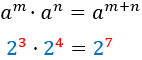Power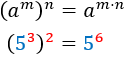Quotient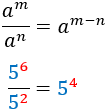Negative exponent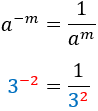Inverse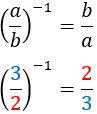Inverse of inverse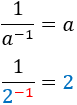## Important Property

The following property will probably be the one we will use the most:

## Product and Quotient of Roots

The product of two roots with the same degree is the root (of same degree) of the product of the radicands, this is,

## $$\sqrt[n]{a}\cdot \sqrt[n]{b} = \sqrt[n]{a\cdot b}$$

The same happens with the quotient:

# Solved Exercises

## Simplify the expressions with fractional exponents

Exercise 1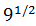Show solution

Exercise 2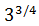Show solution

Exercise 3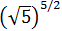Show solution

Exercise 4Show solution

Exercise 5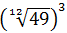Show solution

Exercise 6Show solution

Exercise 7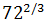Show solution

Exercise 8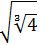Show solution

Exercise 9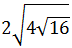Show solution

Exercise 10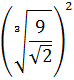Show solution

Exercise 11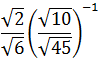Show solution

Exercise 12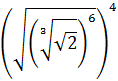Show solution

Exercise 13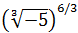Show solution

Exercise 14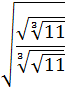Show solution

Exercise 15Show solution